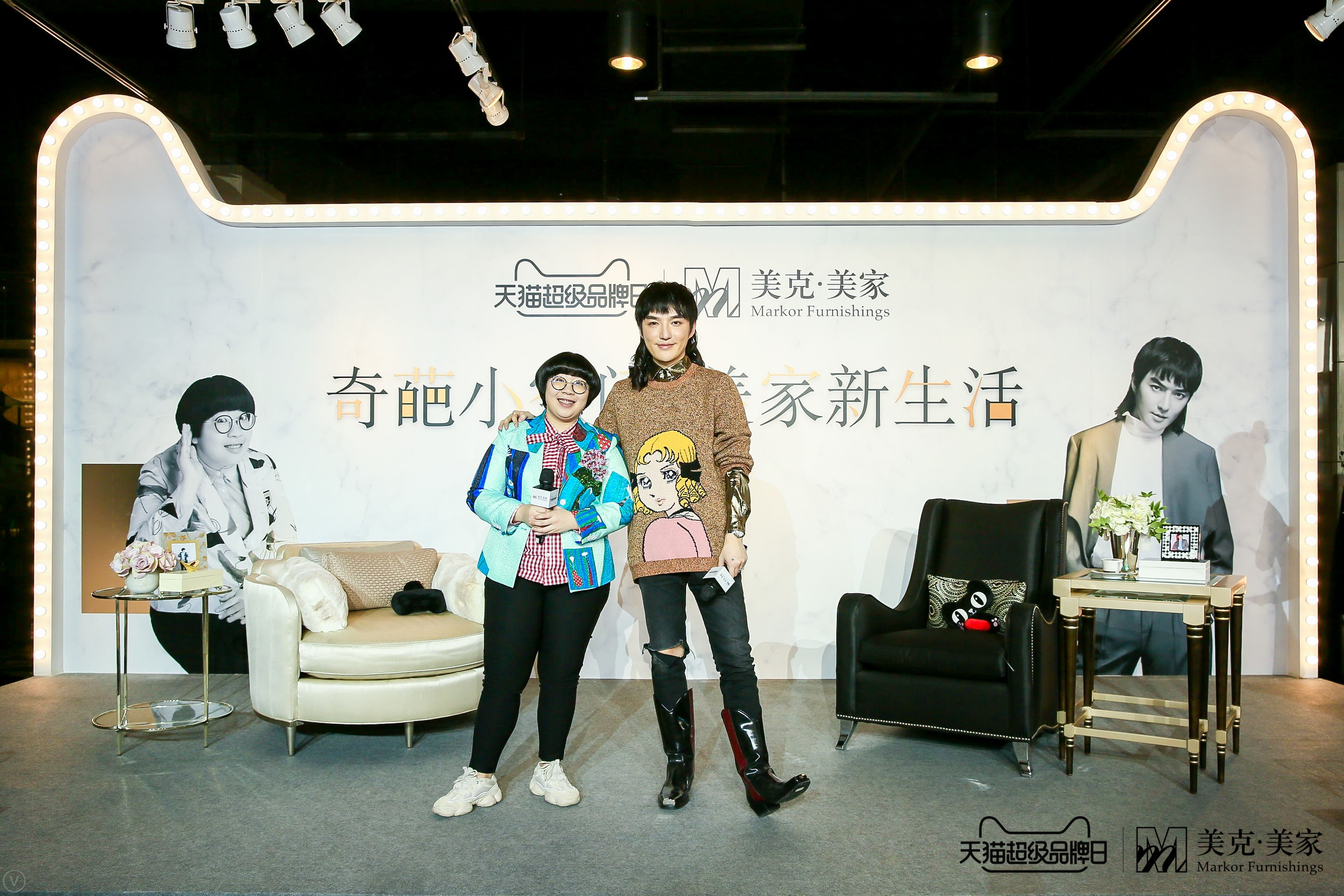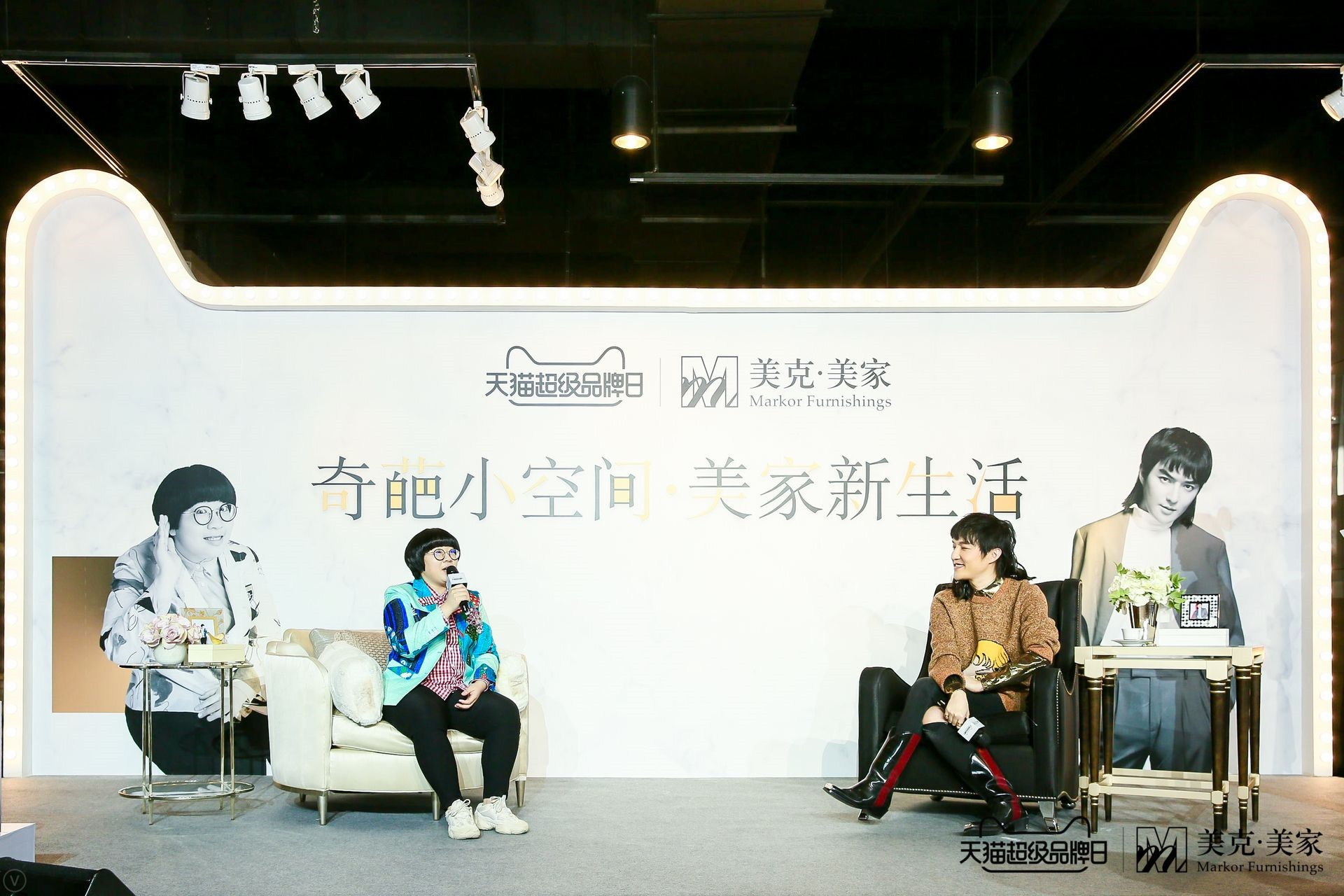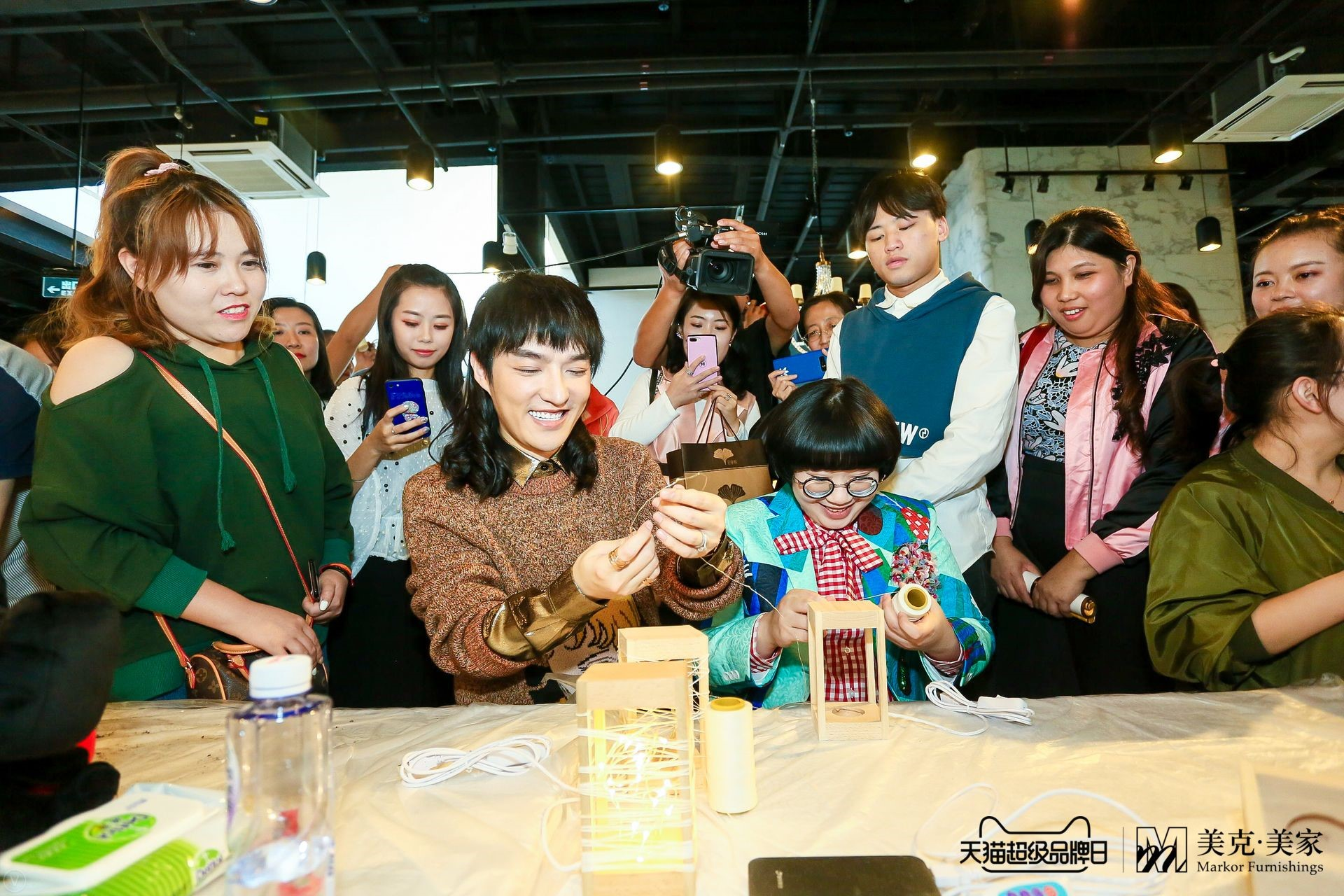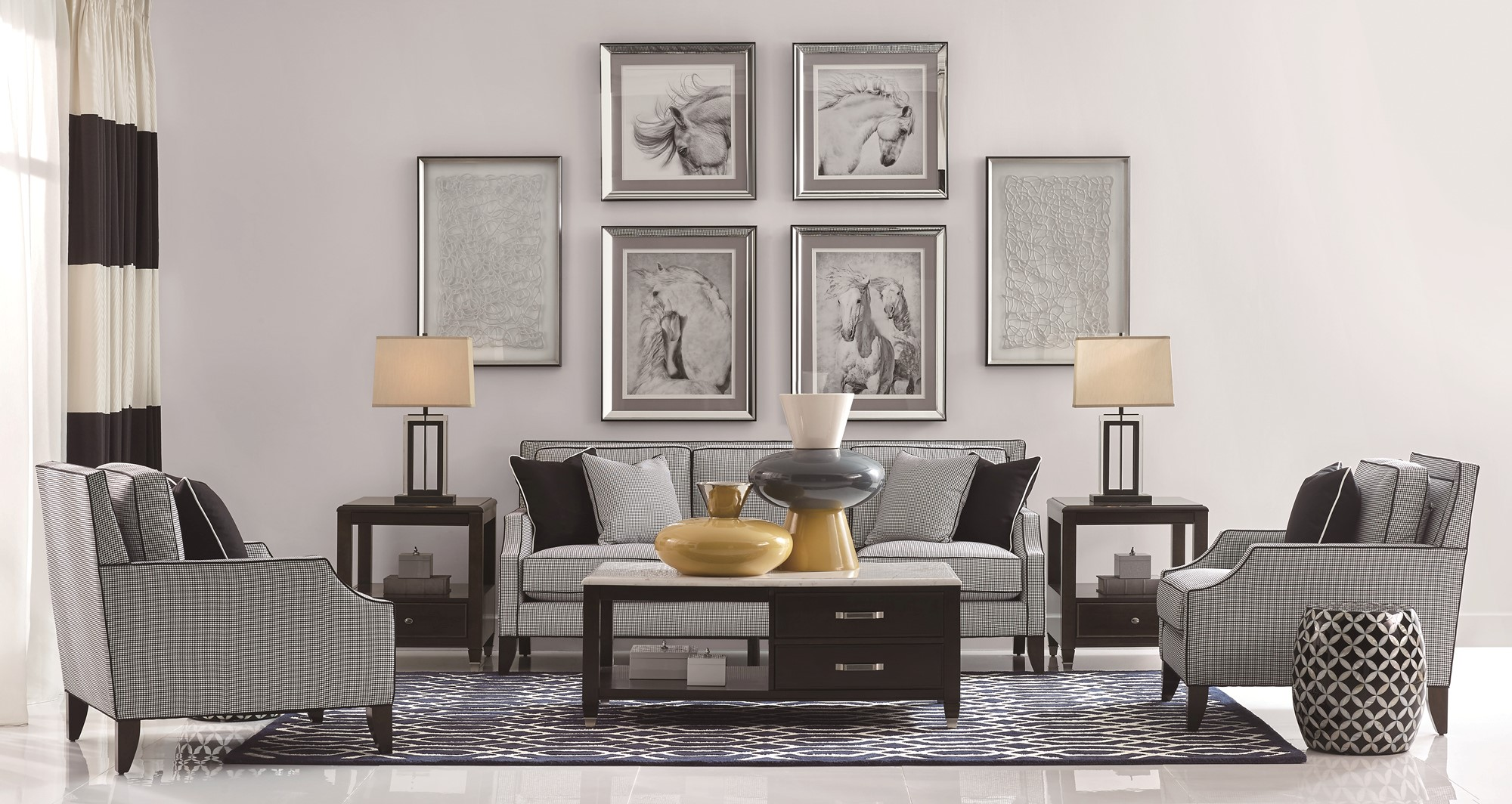|

# 打通设计×消费模式,美克美家缔造小空间新生活

9月23日，美克美家联合天猫在北京丽泽店举办一场以“奇葩小空间 美家新生活”为主题的天猫超级品牌日活动，并在活动现场设置了猫居美家体验区、手工造及3D打印区供消费者体验分享。除此之外还邀请了都市、时尚、娱乐和家居行业的多家媒体，更有超人气“相爱相杀好CP”肖骁和颜如晶惊艳亮相助阵，以精彩纷呈的唇枪舌剑，完美诠释此次美克美家天猫超级品牌日活动的意义，给热爱生活的年轻人带来极致美好的家居生活方式。绝配CP，演绎美家理念“相爱相杀”

在预热悬念视频吊足大家的胃口后，肖骁和颜如晶闪耀登场。众所周知，肖骁与颜如晶以“相爱相杀好闺蜜”的形象深入人心，性格迥异的两人每逢同台必定“短兵相接”，引发最激烈的观点碰撞。活动当天，以“面子派”为代表的肖骁和以“里子派”为代表的颜如晶围绕外观至上还是舒适至上两种截然不同的“美家理念”，展开了激烈辩战。肖骁表示家的概念是分大家和小家，而他和同为单身的颜如晶，现阶段是属于小家的阶段。因此他觉得作为小家，颜值是非常重要的，面子也是非常重要的，小家讲颜值，大家讲舒适，小家不需要有太多的烟火气，不要让大家感受到太多过日子的氛围。颜如晶则与肖骁有不同的见解，她认为每个人都是从小家奔着大家走的，从小才能变大，从单身变成有家庭，所以她认为颜值是时时都在变化的，但合适这个东西从头到尾都是一样的。随后奇袭环节，两人邀请在场媒体与消费者助阵，现场分享他们对于美克美家的认识和理解，以及对于软装理念的思考和选择。最后，让他们“握手言和”的是美克美家。二人一致认同，美克美家秉承“高品位、经典、浪漫、优雅”的理念，以高品位的艺术化家居服务及精致考究的品质追求，为中国城市新中产阶级人群提供国际水准的家居整体解决方案，满足消费者高品位的生活追求，而此次的“奇葩小空间美家新生活”活动，则将优雅、浪漫、经典和高品质的生活方式分享给中小户型的消费者，不仅满足了追求颜值的“面子派”，同时也保证了渴求舒适品质的“里子派”的需求。

辩论结束后，肖骁和颜如晶亲自抽出了六位幸运观众，并送上了美克美家精心准备的咖啡套装。随后二人与现场消费者、媒体一同参与现场手工造DIY，并拍照留念，卖萌、搞怪，充分体现二人“奇葩”一面，让现场气氛更加热烈。引领行业趋势 贴心打造天猫超级品牌日

此次“奇葩小空间 美家新生活”是美克美家为天猫超级品牌日精心筹备与打造的主题活动。超级品牌日期间，美克美家推出一对一设计师3D软装设计服务：通过一对一贴心服务，明确把握每一位消费者的风格意向，从了解需求到搭配再到3D设计效果，比拟真实的场景呈现，来满足消费者对美好生活方式的诉求。2014年美克美家成立电子商务部正式运营电商业务，之后仅用3年的时间实现店铺营收破亿元，天猫逐渐成为美克美家品牌营销的主阵地。美克美家天猫旗舰店的成功，得益于对年轻消费群体需求的精准把控，并依托平台大数据优势，日益精确地了解线上消费顾客的特质——对生活方式有独特见解，同时注重生活品质的新型中产用户，从而为其提供更加舒适购物体验。

随着消费升级，年轻消费群体对家居的诉求，已经从单一地追求简单实用性，进而演变到现在的情感性，家居承载着他们对家和生活最直接的情感表达。根植于中产阶级年轻化和消费升级的大背景，美克美家深深植入品牌精神、专注生活方式的现代化家居理念，恰恰契合当今年轻人追求个性，在消费中寻求自我主张表达的生活方式。美克美家以“高品位、经典、浪漫、优雅”为价值主张，主导家居产品极致考究的设计，和全方位提升的服务体系，将消费者对家的美学梦想，升华成当代艺术化家居生活方式的高雅体验，让消费者在家居空间高格调的积极创造中，享受高度契合其切身需求的生活方式，呼应当下年轻消费者对居家的美好诉求。

软装模式新升级聚焦艺术生活方式

此次美克美家天猫超级品牌日期间，美克美家通过对线上消费者及近年购房户型需求的洞察，针对一批预算较低但向往美好生活的线上消费者，以“小空间”为核心逻辑，将不同特点的系列产品充分结合当下流行的美好生活方式，推出“6个中小户型套餐，6种生活方式”，六大套系产品分别包括马赛晨光、查尔斯汀、新鸢尾乡旅、邦迪海滩、时尚变奏、公园大道。

活动当日的互动区域，美克美家为参观者提供了沉浸式体验，所采用的正是沿袭其一贯的时尚与风格“邦迪海滩”套系产品。精良选材与精致做工在设计师的巧思与细节中相得益彰，细腻考究的整体外观契合了当下流行趋势，尽显创意光芒——线条简洁流畅，仿若邦迪海滩绵延的海岸线，焦糖棕Cersue涂装工艺仿若浓郁咖啡，增加了视觉的层次感和暖度，量身设计的定制拉手，让人联想到海滩边惬意的鸡尾酒吧。一直以来，美克美家致力于生活方式研究，将现代化家居理念深深植入品牌精神，专注生活方式的设计、整合，提供集设计服务、家具产品、软装配饰、家居用品为一体的家居整体服务，以全球化的视野和专业化的考量，和严格乃至严苛的工艺态度，积极践行生活的艺术，帮助更多的消费者实现艺术的生活。美克美家贩卖的不仅是家居产品，更是一种艺术情怀，一种美学生活方式。此次亮相天猫超级品牌日的六大套系，亦以其历经时间沉淀的美好设计，惊艳消费者。

今年三月，美克美家品牌代言人靳东一同掀开品牌战略升级大幕，提出“开启软装新模式，享受艺术新生活”的品牌主张，并据此策动、展开一场精彩纷呈的美家之旅，引领家居行业翻开品质升级的崭新篇章。美克美家顺应时代潮流，结合电商这种年轻人最习以为常的消费方式，推出超级品牌日活动，为消费者悉力打造全渠道、跨场景、全终端、全链路无缝的消费体验，让更多人可以体验到美克美家为生活带来的更多种美好的可能。

审美的视角是独特的，但美却存在着共性。美克美家天猫超级品牌日，为这句话做出了最好的诠释。

`声明：本文由入驻焦点开放平台的作者撰写，除焦点官方账号外，观点仅代表作者本人，不代表焦点立场错误信息举报电话： 400-099-0099，邮箱：jubao@vip.sohu.com，或点此进行意见反馈，或点此进行举报投诉。`A B C D E F G H J K L M N P Q R S T W X Y Z
A - B - C - D - E
• A
• 鞍山
• 安庆
• 安阳
• 安顺
• 安康
• 澳门
• B
• 北京
• 保定
• 包头
• 巴彦淖尔
• 本溪
• 蚌埠
• 亳州
• 滨州
• 北海
• 百色
• 巴中
• 毕节
• 保山
• 宝鸡
• 白银
• 巴州
• C
• 承德
• 沧州
• 长治
• 赤峰
• 朝阳
• 长春
• 常州
• 滁州
• 池州
• 长沙
• 常德
• 郴州
• 潮州
• 崇左
• 重庆
• 成都
• 楚雄
• 昌都
• 慈溪
• 常熟
• D
• 大同
• 大连
• 丹东
• 大庆
• 东营
• 德州
• 东莞
• 德阳
• 达州
• 大理
• 德宏
• 定西
• 儋州
• 东平
• E
• 鄂尔多斯
• 鄂州
• 恩施
F - G - H - I - J
• F
• 抚顺
• 阜新
• 阜阳
• 福州
• 抚州
• 佛山
• 防城港
• G
• 赣州
• 广州
• 桂林
• 贵港
• 广元
• 广安
• 贵阳
• 固原
• H
• 邯郸
• 衡水
• 呼和浩特
• 呼伦贝尔
• 葫芦岛
• 哈尔滨
• 黑河
• 淮安
• 杭州
• 湖州
• 合肥
• 淮南
• 淮北
• 黄山
• 菏泽
• 鹤壁
• 黄石
• 黄冈
• 衡阳
• 怀化
• 惠州
• 河源
• 贺州
• 河池
• 海口
• 红河
• 汉中
• 海东
• 怀来
• I
• J
• 晋中
• 锦州
• 吉林
• 鸡西
• 佳木斯
• 嘉兴
• 金华
• 景德镇
• 九江
• 吉安
• 济南
• 济宁
• 焦作
• 荆门
• 荆州
• 江门
• 揭阳
• 金昌
• 酒泉
• 嘉峪关
K - L - M - N - P
• K
• 开封
• 昆明
• 昆山
• L
• 廊坊
• 临汾
• 辽阳
• 连云港
• 丽水
• 六安
• 龙岩
• 莱芜
• 临沂
• 聊城
• 洛阳
• 漯河
• 娄底
• 柳州
• 来宾
• 泸州
• 乐山
• 六盘水
• 丽江
• 临沧
• 拉萨
• 林芝
• 兰州
• 陇南
• M
• 牡丹江
• 马鞍山
• 茂名
• 梅州
• 绵阳
• 眉山
• N
• 南京
• 南通
• 宁波
• 南平
• 宁德
• 南昌
• 南阳
• 南宁
• 内江
• 南充
• P
• 盘锦
• 莆田
• 平顶山
• 濮阳
• 攀枝花
• 普洱
• 平凉
Q - R - S - T - W
• Q
• 秦皇岛
• 齐齐哈尔
• 衢州
• 泉州
• 青岛
• 清远
• 钦州
• 黔南
• 曲靖
• 庆阳
• R
• 日照
• 日喀则
• S
• 石家庄
• 沈阳
• 双鸭山
• 绥化
• 上海
• 苏州
• 宿迁
• 绍兴
• 宿州
• 三明
• 上饶
• 三门峡
• 商丘
• 十堰
• 随州
• 邵阳
• 韶关
• 深圳
• 汕头
• 汕尾
• 三亚
• 三沙
• 遂宁
• 山南
• 商洛
• 石嘴山
• T
• 天津
• 唐山
• 太原
• 通辽
• 铁岭
• 泰州
• 台州
• 铜陵
• 泰安
• 铜仁
• 铜川
• 天水
• 天门
• W
• 乌海
• 乌兰察布
• 无锡
• 温州
• 芜湖
• 潍坊
• 威海
• 武汉
• 梧州
• 渭南
• 武威
• 吴忠
• 乌鲁木齐
X - Y - Z
• X
• 邢台
• 徐州
• 宣城
• 厦门
• 新乡
• 许昌
• 信阳
• 襄阳
• 孝感
• 咸宁
• 湘潭
• 湘西
• 西双版纳
• 西安
• 咸阳
• 西宁
• 仙桃
• 西昌
• Y
• 运城
• 营口
• 盐城
• 扬州
• 鹰潭
• 宜春
• 烟台
• 宜昌
• 岳阳
• 益阳
• 永州
• 阳江
• 云浮
• 玉林
• 宜宾
• 雅安
• 玉溪
• 延安
• 榆林
• 银川
• Z
• 张家口
• 镇江
• 舟山
• 漳州
• 淄博
• 枣庄
• 郑州
• 周口
• 驻马店
• 株洲
• 张家界
• 珠海
• 湛江
• 肇庆
• 中山
• 自贡
• 资阳
• 遵义
• 昭通
• 张掖
• 中卫

1室1厅1厨1卫1阳台

1
2
3
4
5

0
1
2

1

1

0
1
2
3报名成功，资料已提交审核A B C D E F G H J K L M N P Q R S T W X Y Z
A - B - C - D - E
• A
• 鞍山
• 安庆
• 安阳
• 安顺
• 安康
• 澳门
• B
• 北京
• 保定
• 包头
• 巴彦淖尔
• 本溪
• 蚌埠
• 亳州
• 滨州
• 北海
• 百色
• 巴中
• 毕节
• 保山
• 宝鸡
• 白银
• 巴州
• C
• 承德
• 沧州
• 长治
• 赤峰
• 朝阳
• 长春
• 常州
• 滁州
• 池州
• 长沙
• 常德
• 郴州
• 潮州
• 崇左
• 重庆
• 成都
• 楚雄
• 昌都
• 慈溪
• 常熟
• D
• 大同
• 大连
• 丹东
• 大庆
• 东营
• 德州
• 东莞
• 德阳
• 达州
• 大理
• 德宏
• 定西
• 儋州
• 东平
• E
• 鄂尔多斯
• 鄂州
• 恩施
F - G - H - I - J
• F
• 抚顺
• 阜新
• 阜阳
• 福州
• 抚州
• 佛山
• 防城港
• G
• 赣州
• 广州
• 桂林
• 贵港
• 广元
• 广安
• 贵阳
• 固原
• H
• 邯郸
• 衡水
• 呼和浩特
• 呼伦贝尔
• 葫芦岛
• 哈尔滨
• 黑河
• 淮安
• 杭州
• 湖州
• 合肥
• 淮南
• 淮北
• 黄山
• 菏泽
• 鹤壁
• 黄石
• 黄冈
• 衡阳
• 怀化
• 惠州
• 河源
• 贺州
• 河池
• 海口
• 红河
• 汉中
• 海东
• 怀来
• I
• J
• 晋中
• 锦州
• 吉林
• 鸡西
• 佳木斯
• 嘉兴
• 金华
• 景德镇
• 九江
• 吉安
• 济南
• 济宁
• 焦作
• 荆门
• 荆州
• 江门
• 揭阳
• 金昌
• 酒泉
• 嘉峪关
K - L - M - N - P
• K
• 开封
• 昆明
• 昆山
• L
• 廊坊
• 临汾
• 辽阳
• 连云港
• 丽水
• 六安
• 龙岩
• 莱芜
• 临沂
• 聊城
• 洛阳
• 漯河
• 娄底
• 柳州
• 来宾
• 泸州
• 乐山
• 六盘水
• 丽江
• 临沧
• 拉萨
• 林芝
• 兰州
• 陇南
• M
• 牡丹江
• 马鞍山
• 茂名
• 梅州
• 绵阳
• 眉山
• N
• 南京
• 南通
• 宁波
• 南平
• 宁德
• 南昌
• 南阳
• 南宁
• 内江
• 南充
• P
• 盘锦
• 莆田
• 平顶山
• 濮阳
• 攀枝花
• 普洱
• 平凉
Q - R - S - T - W
• Q
• 秦皇岛
• 齐齐哈尔
• 衢州
• 泉州
• 青岛
• 清远
• 钦州
• 黔南
• 曲靖
• 庆阳
• R
• 日照
• 日喀则
• S
• 石家庄
• 沈阳
• 双鸭山
• 绥化
• 上海
• 苏州
• 宿迁
• 绍兴
• 宿州
• 三明
• 上饶
• 三门峡
• 商丘
• 十堰
• 随州
• 邵阳
• 韶关
• 深圳
• 汕头
• 汕尾
• 三亚
• 三沙
• 遂宁
• 山南
• 商洛
• 石嘴山
• T
• 天津
• 唐山
• 太原
• 通辽
• 铁岭
• 泰州
• 台州
• 铜陵
• 泰安
• 铜仁
• 铜川
• 天水
• 天门
• W
• 乌海
• 乌兰察布
• 无锡
• 温州
• 芜湖
• 潍坊
• 威海
• 武汉
• 梧州
• 渭南
• 武威
• 吴忠
• 乌鲁木齐
X - Y - Z
• X
• 邢台
• 徐州
• 宣城
• 厦门
• 新乡
• 许昌
• 信阳
• 襄阳
• 孝感
• 咸宁
• 湘潭
• 湘西
• 西双版纳
• 西安
• 咸阳
• 西宁
• 仙桃
• 西昌
• Y
• 运城
• 营口
• 盐城
• 扬州
• 鹰潭
• 宜春
• 烟台
• 宜昌
• 岳阳
• 益阳
• 永州
• 阳江
• 云浮
• 玉林
• 宜宾
• 雅安
• 玉溪
• 延安
• 榆林
• 银川
• Z
• 张家口
• 镇江
• 舟山
• 漳州
• 淄博
• 枣庄
• 郑州
• 周口
• 驻马店
• 株洲
• 张家界
• 珠海
• 湛江
• 肇庆
• 中山
• 自贡
• 资阳
• 遵义
• 昭通
• 张掖
• 中卫• 手机• 分享
• 设计
免费设计
• 计算器
装修计算器
• 入驻
合作入驻
• 联系
联系我们
• 置顶
返回顶部